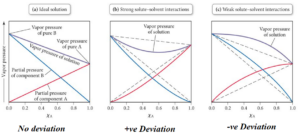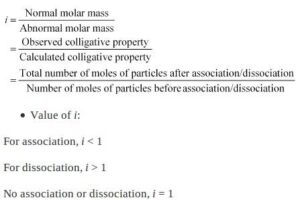# Chapter Solutions Important PointsChapter Solutions Important Points

Knowledge Points

1. Solutions are the homogeneous mixtures of two or more than two components.

2. Binary Solution: A solution having two components is called a binary solution. Components of a binary solution are solute and solvent.

3. When the solvent is in solid-state, the solution is called a solid solution.

4. When the solvent is in liquid state, solution is called liquid solution.

5. When the solvent is in gaseous state, solution is called gaseous solution.

6. Concentration is the amount of solute in given amount of solution.

7. Mass by volume percentage (w/v): Mass of the solute dissolved in100 mL of solution.

8. Molality (m) is the number of moles of solute present in 1kg of solvent.

9. Molarity (M) is the number of moles of solute present in 1L of solution.

10. Normality (N) is the number of gram equivalent of solute dissolved per litre of solution.

Chapter Solutions Important Points

11. Solubility is its maximum amount that can be dissolved in a specified amount of solvent at a specified temperature.

12. A solution in which no more solute can be dissolved at the same temperature and pressure is called a saturated solution.

13. In a nearly saturated solution if the dissolution process is an endothermic process, solubility increases with increase in temperature.

14. In a nearly saturated solution if the dissolution process is an exothermic process, solubility decreases with increase in temperature.

15. Henry’s Law: The law states at a constant temperature the solubility of a gas in a liquid is directly proportional to the pressure of the gas. Henry’s law can also be stated as the partial pressure of the gas in the vapour phase is proportional to the mole fraction of the gas in the solution.
P = KH x

16. When a non-volatile solute is dissolved in a volatile solvent, the vapour pressure of the solution is less than that of pure solvent.

17. According to Raoult’s law for a solution of volatile liquids the partial vapour pressure of each component in the solution is directly proportional to its mole fraction.
p1 = po1 x1
p2 = po2 x2
Using Dalton’s law of partial pressure the total pressure of the solution is calculated.

18. On comparison of Raoult’s law and Henry’s law, it is observed thatthe partial pressure of volatile component or gas is directly proportional to its mole fraction in solution. In the case of Henry’s Law, the proportionality constant is KHand it is different from p0  which is the partial pressure of pure component. Raoult’s Law becomes a special case of Henry’s Law when KH becomes equal to  p0in Henry’s law.

19. Liquid–liquid solutions can be classified into ideal and non-ideal solutions on basis of Raoult’s Law.

 Ideal solutions Non- ideal solutions The solutions that obey Raoult’s Law over the entire range of concentrations are known as ideal solutions. When a solution does not obey Raoult’s Law over the entire range of concentration, then it is called a non-ideal solution. △H mix = 0 and △mixV = 0 △H mix = 0 and △mixV = 0 The intermolecular attractive forces between solute molecules and solvent molecules are nearly equal to those present between solute and solvent molecules i.e. A-A and B-B interactions are nearly equal to those between A-B The intermolecular attractive forces between solute molecules and solvent molecules are not equal to those present between solute and solvent molecules i.e. A-A and B-B interactions are not equal to those between A-B

20. Non- ideal solutions are of two types:

 Non-ideal solution showing positive deviation Non-ideal solution showing negative deviation The vapour pressure of a solution is higher than that predicted by Raoult’s Law The vapour pressure of a solution is lower than that predicted by Raoult’s Law The intermolecular attractive forces between solute-solvent molecules are weaker than those between solute-solute and solvent-solvent molecules i.e.A-B < A-A and B-B interactions The intermolecular attractive forces between solute-solvent molecules are stronger than those between solute-solute and solvent-solvent molecules i.e. A-B > A-A and B-B interactionsChapter Solutions Important Points

21. Azeotropes are binary mixtures having the same composition in the liquid and vapour phase and boil at a constant temperature. Liquids forming azeotrope cannot be separated by fractional distillation.

22. There are two types of azeotropes called minimum boiling azeotrope and maximum boiling azeotrope.

23. The solutions which show a large positive deviation from Raoult’s law form minimum boiling azeotrope at a specific composition.

24. The solutions that show large negative deviation from Raoult’slaw form maximum boiling azeotrope at a specific composition.

25. Properties of solution which depends on only the number of solute particles but not on the nature of solute are called colligative properties.

26. There are four colligative properties:
(a) Relative lowering of vapour pressure
(b) Elevation of boiling point
(c) Depression of freezing point
(d) Osmotic pressure

27. Relative lowering of vapour pressure: Difference in the vapour pressure of pure solvent (o)and solution (p1) represents lowering in vapour pressure (o– p1). Dividing lowering in vapour pressure by the vapour pressure of the pure solvent is called relative lowering of vapour pressure.

28. Elevation of the boiling point: The difference in boiling points of solution (Tb) and pure solvent ( T 0 ) is called elevation in boiling point.

29. Depression of freezing point: The lowering of the vapour pressure of solution causes a lowering of a freezing point compared to that of the pure solvent. The difference in freezing point of the pure solvent ( Tf0) and solution ( Tf ) is called the depression in freezing point.

30. For a dilute solution depression in freezing point is a colligative property because it is directly proportional to molal concentration of solute.

Chapter Solutions Important Points

31. Osmosis: The phenomenon of flow of solvent molecules through a semi-permeable membrane from pure solvent to the solution is called Osmosis.

32.Osmotic pressure: The excess pressure that must be applied to the solution to prevent the passage of solvent into the solution through a semi-permeable membrane is called osmotic. Osmotic pressure is a colligative property as it depends on the number of solute particles and not on their identity. For a dilute solution, osmotic pressure (π) is directly proportional to the concentration (C) of the solution i.e. 𝜋  CRT

33. Osmotic pressure can also be used to determine the molar mass of solute using the equation
M2 =  w2RT/Pi V

34. Two solutions having the same osmotic pressure at a given temperature are called an isotonic solution.

35. If a solution has more osmotic pressure than other solution it is called a hypertonic solution.

36. If a solution has less osmotic pressure than other solution it is called a hypotonic solution.

37. Reverse osmosis: The process of movement of a solvent through a semi-permeable membrane from the solution to the pure solvent by applying excess pressure on the solution side is called reverse osmosis.

38. Colligative properties help in the calculation of the molar mass of solutes.

39. Molar mass that is either lower or higher than expected or normal molar mass is called abnormal molar mass.

40. van’t Hoff factor (i) accounts for the extent of dissociation or association. Value of i is less than unity in case solute undergo association Value of i is greater than unity in case solute undergo dissociation.Chapter Solutions Important Points